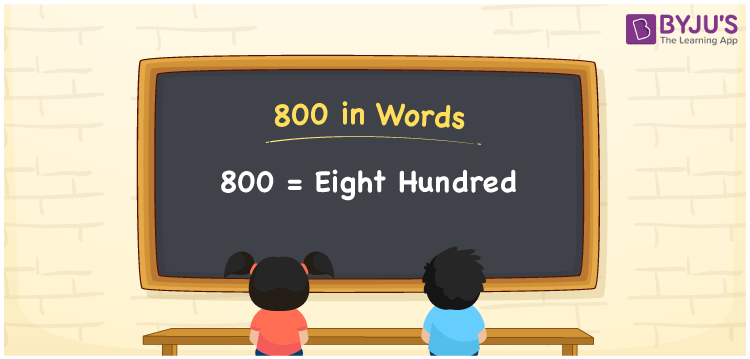# 800 in Words

800 in words is written as “Eight hundred”. In Mathematics, numbers are used to represent the value of something. 800 is a cardinal number that expresses quantity. If the price of a bat is Rs.800, then it can be represented as Rupees Eight hundred, in English. Learn more about Numbers In Words at BYJU’S.

 800 in Words Eight hundred Eight hundred in Numbers 800

## 800 in English Words

800 in English Words is expressed as given below:## How to Write 800 in Words?

The number 800 in words can be written using a place value system, where we can identify the position of each digit in the number. Since, 800 is a three-digit number, thus, we can place each digit in the given table, such that;

 Hundreds Tens Ones 8 0 0

From the above table, 8 is at hundred place, 0 is at tens place and another 0 is at ones place. We need to read the number from right to left.

Ones>Tens>Hundreds

Hence, 8 comes at a hundred place so together we can write the number as Eight hundred.

### Expanded Form of 800

We can write the expanded form as:

8 × Hundred + 0 × Ten + 0 × One

= 8 × 100 + 0 × 10 + 0 × 1

= 800

= Eight hundred

800 is a whole number that is succeeded by 799 and preceded by 801. Learn more about the number 800 below:

• 800 in Words – Eight hundred
• Is 800 an odd number? – No
• Is 800 an even number? – Yes
• Is 800 a perfect square number? – No
• Is 800 a perfect cube number? – No
• Is 800 a prime number? – No
• Is 800 a composite number? – Yes

## Frequently Asked Questions on 800 in words

Q1

### What is 800 in words?

800 in words is given by Eight hundred.
Q2

### What is the rule to write 800 in words?

800 in words is written based on the place values of each digit. 800 is thee digit number, where 8 is at hundreds place and the two zeros are at tens and ones place respectively. Thus, reading from right to left, we can express 800 as Eight hundred.
Q3

### What is the value of 800 + 100 in words?

800 + 100 = 900, i.e., Nine hundred in words